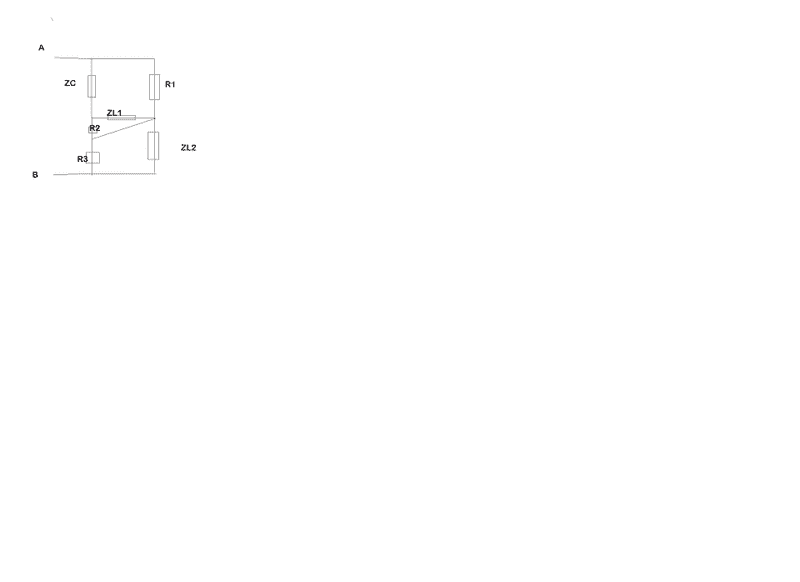# Equivalent impedance

## Homework Statement

Hi everyone, I'm just having a little trouble with equivalent resistance in a bridge, in the image I attached. The textbook says that the way to solve this is to put R3 and ZL2 in series, that series is in parallel to the short circuit in diagonal, and then at the end we have a parallel between:

1 Zc

2 The series between R1 and the parallel zl1 and R2.

The point is, I can't understand why it puts zl2 and r3 in series, when they're divided by a clamp.

2. Homework Equations

## The Attempt at a Solution

My reasoning was, instead, to put R3 in parallel with zl2. We have a parallel also between Zl1 and R2. Then we solve the circuit with the usual methodsLast edited by a moderator:

•